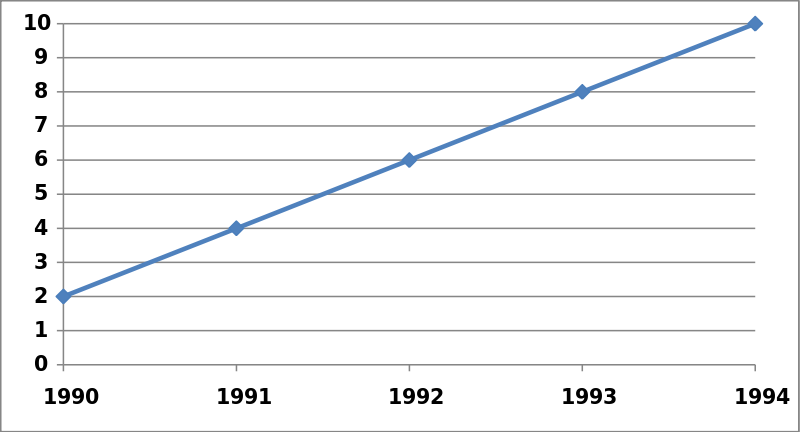Back

# Decrypting Cryptography

I was particularly excited to write about cryptanalysis this month because I was fascinated with secret messages, spys, and the like when I was young. Encyclopedia Brown, Nancy Drew, lemon juice ink, pig latin, secret codes… I loved it all. With computers’ ability to solve equations at mind blowing speeds, software encryption goes beyond just replacing letters with numbers to create a code- way, way beyond.

# Cryptography Basics

Just reading about cryptography can be cryptic so let’s begin with some basic vocabulary.

Plaintext– The is the original message or information that you want to change. The “decrypted” text
Ciphertext– The resulting changed message. The “encrypted” text.
Encrypt– Turning plaintext into ciphertext.
Decrypt– Turning ciphertext into plaintext.
Cipher– The algorithm that is applied to the plaintext to encrypt it.

So what is cryptography? It is using a cipher to encrypt plaintext into ciphertext. What sort of information gets encrypted? Passwords, credit card information, emails, website traffic, documents, personal identification information such as names, addresses, social security numbers, etc. all can, and should, be digitally encrypted. Cryptanalysis is analyzing ciphers, plaintext, and ciphertext to extract useful information or break the cipher.

# Statistically Speaking

Much of cryptography is based on statistical analysis. Is it more likely you could guess the exact two letter word I am thinking of or the five letter word? What’s the maximum number of tries it would take? What’s the likelihood that you would get it on the first try?

# What Makes a Good Cipher?

A good cipher needs to have both confusion and diffusion. Confusion is the ability for a cipher’s output to be non-linear. I think of it like a line graph.Even though it doesn’t show what happened in 1995 or when 12 happened, we can still extrapolate that in 1995, there were 12. If this were less linear and the data points were all over the place, it would be much harder to know what happened in 1995, even if it did follow a pattern.

Diffusion describes a cipher’s ability to change a large portion of the output when only one item is changed. For instance, if CAT= RWO and BAT= NWO, you could deduce that A=W and T=O. However, if your cipher spit out CAT =RWO and BAT = FAL, figuring out what A and T are would be exponentially more difficult.

# Cryptographic Attacks

So now that you have some basic understanding of what cryptopgraphy is and what makes a good cipher, I’ll get into a few different cryptographic attacks.

## Brute Force

The most straightforward way to break a cipher is to brute force it. You take what you know and then fill in the blanks with every possible combination until you find it. It is tedious and time consuming, but it does work.

For instance, imagine I give you a number and you apply a formula to that number and respond with the answer. By hammering you with questions, I can work out the formula. If I say 1, you say 2. I say 2, you say 4. 3, 6. 4, 8. In this case, the formula is multiplying the input number by 2.

## Plaintext Attacks and Cipherbased Attack Methods

These start based on the information given and then become more refined based on the results. When all a hacker has access to is the plaintext or ciphertext, that is called a known plaintext or ciphertext only attack, respectively. Chosen plaintext attacks occur when the cryptanalyst inserts their own chosen plaintext into the cipher and then studies the output. The chosen ciphertext method is almost an inverse and involves choosing some ciphertext to then find the resulting plaintext. Lastly, the adaptive chosen ciphertext method builds upon results from the previous methods. The analyst “adapts” which ciphertext or plaintext to examine based on their previous results.

## Meet-in-the Middle Attack

This sort of attack is useful for double encryption. Double encryption is just what it sounds like; encrypt the plaintext and then encrypt it again. To use a meet-in-the-middle attack, the analyst first brute forces the first encryption and makes a table of the results. Then they brute force decrypt the ciphertext and compare the results to the table. When a result matches, the analyst has the cipher for each encryption step.

abc=yeh=fyt
Solve for (abc=yeh). Solve for (fyt=yeh). Compare the results!

## Birthday Attacks

This one is a little harder to wrap your head around. Heck, just the paradox it’s based on feels counterintuitive. The birthday paradox states that in a set of 23 randomly chosen people, there is a 50/50 chance two of them will have the same birthday.

Birthday attacks are best for ciphers such as hashing algorithms that are equally likely to output any one of a set of values. A birthday attack makes use of this and looks for two inputs that give the same value. This becomes useful for deceptive maneuvers. Information can be changed with just enough similar parts to have the same hash value as the original but in reality be a fraud.

# Linear and Differential Cryptanalysis

Linear and differential cryptanalysis looks for patterns in multiple sets of plaintext and ciphertext. Linear cryptanalysis evaluates the pairs for similarities that could be plotted linearly. Using this extrapolated linear formula, subsequent iterations in breaking the cipher are more accurate. Differential cryptanalysis works similarly, but evaluates the pairs by their differences.

# Conclusion

Cryptography is full of mathematical equations and statistics. Like you would with a math equation, analysts take the information they are given and work the problem until they break the cipher or obtain what they are looking for.